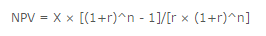Accounting

# What is Discounted Cash Flow?

Discounted cash flow is a technique that determines the present value of future cash flows. Under the method, one applies a discount rate to each periodic cash flow that is derived from an entity’s cost of capital. Multiplying this discount by each future cash flow results in an amount that is, in aggregate, the present value of all future cash flows.

By calculating the discounted cash flows for a number of different investment choices, one can select the alternative that results in the greatest discounted cash flows. This concept is useful for calculating the value of a prospective acquisition, of a possible annuity investment, or of a fixed asset purchase.

The foundation of discounted cash flow analysis is the concept that cash received today is more valuable than cash received at some point in the future. The reason is that someone who agrees to receive payment at a later date foregoes the ability to invest that cash right now. The only way for someone to agree to a delayed payment is to pay them for the privilege, which is known as interest income.

For example, if a person owns \$10,000 now and invests it at an interest rate of 10%, then she will have earned \$1,000 by having use of the money for one year. If she were instead to not have access to that cash for one year, then she would lose the \$1,000 of interest income. The interest income in this example represents the time value of money.

Two analysis methods that employ the discounted cash flow concept are net present value and the internal rate of return, which are described next.

Net Present Value

Net present value (NPV) analysis is useful for determining the current value of a stream of cash flows that extend out into the future. It can also be used to compare several such cash flows to decide which has the largest present value. NPV is commonly used in the analysis of capital purchasing requests, to see if an initial payment for fixed assets and other expenditures will generate net positive cash flows.

To calculate net present value, we use the following formula:Where:

X = The amount received per period
n = The number of periods
r = The rate of return

Internal Rate of Return

The internal rate of return (IRR) is the rate of return at which the present value of a series of future cash flows equals the present value of all associated costs. IRR is commonly used in capital budgeting to discern the rate of return on the estimated cash flows arising from an expected investment. The project having the highest IRR is selected for investment purposes.

The easiest way to calculate the internal rate of return is to open Microsoft Excel and then follow these steps:

• Enter in any cell a negative figure that is the amount of cash outflow in the first period. This is normal when acquiring fixed assets, since there is an initial expenditure to acquire and install the asset.
• Enter the subsequent cash flows for each period following the initial expenditure in the cells immediately below the cell where the initial cash outflow figure was entered.
• Access the IRR function and specify the cell range into which you just made entries. The internal rate of return will be calculated automatically. It may be useful to use the Increase Decimal function to increase the number of decimal places appearing in the calculated internal rate of return.

As an example, a company is reviewing a possible investment for which there is an initial expected investment of \$20,000 in the first year, followed by incoming cash flows of \$12,000, \$7,000 and \$4,000 in the next three years. If you input this information into the Excel IRR function, it returns an IRR of 8.965%.